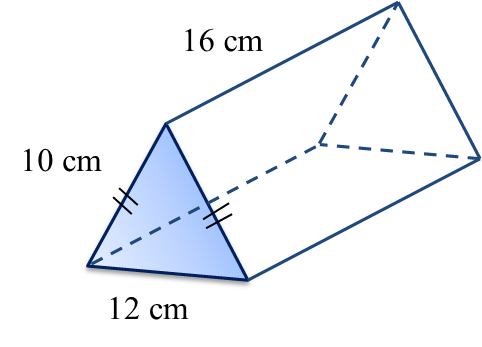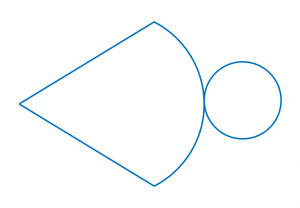# 6.5.2 Volume of Three Dimensional Shapes, PT3 Practice

 
 
Question 4:
 
The cross section of the prism shown is an isosceles triangle.The volume of the prism, in cm3, is
 
 

  Solution:
    $\begin{array}{l}\text{Height of the}△=\sqrt{{10}^{2}-{6}^{2}}\\ \text{}=\sqrt{64}\\ \text{}=8cm\\ \\ \text{Volume of prism}\\ =\text{Area of cross section}×\text{Length}\\ =\left(\frac{1}{\overline{)2}}×{\overline{)12}}^{6}×8\right)×16\\ =768c{m}^{3}\end{array}$

 

 
 
 
 
Question 5:
 
A right circular cone has a volume of 77 cm3 and a circular base of radius 3.5 cm. Calculate its height.
 
 

  Solution:
   

 

 

Question 6:
Puan Rafidah wants to prepare a right circular cone shaped container in order to fill it with candies for her family Hari Raya Celebration. Diagram below shows the net of a right circular cone.The circumference of the lid is 44 cm and its height is 24 cm.
Calculate the volume, in cm3 , of the container.
$\left[\pi =\frac{22}{7}\right]$

Solution:

Question 7:
A barrel contains 36 l of mineral water. ⅔ of the mineral water is mixed with 6 bottles of syrup, with a volume of 1.5 l each. The drink will be poured into a number of bottles with the same volume of 300 ml.
How many number of bottles needed?

Solution: